﻿ Al/Bi2O3复合材料反应燃烧特性的数值分析 Numerical Simulation of Combustion Char-acteristics of Nanothermite Al/Bi2O3

Material Sciences
Vol.07 No.08(2017), Article ID:22817,10 pages
10.12677/MS.2017.78093

Numerical Simulation of Combustion Characteristics of Nanothermite Al/Bi2O3

Haiyang Yu, Yajun Wang*, Liang Guo

State Key Laboratory of Explosion Science and Technology, Beijing Institute of Technology, BeijingReceived: Nov. 5th, 2017; accepted: Nov. 19th, 2017; published: Nov. 27th, 2017ABSTRACT

To study combustion characteristics of nanothermite Al/Bi2O3, a one-dimensional combustion model was established based on solid heat-transfer, combustion theory and chemical kinetics. By using COMSOL Multiphysics, combustion characteristics of nanothermite Al/Bi2O3 were simulated. The results show that there are three steps in the reaction, including heating up before combustion, diffusion of combustion and local thermodynamic equilibrium. The Al/Bi2O3 with stoichiometric ratio (2:1) has the best combustion characteristics. The highest equilibrium temperature, reaction rate and combustion velocity are 4217 K, 1.36 × 107 s−1 and 671.6 m/s respectively. With the decrease of particle size and activation energy, equilibrium temperature decreased, but the reaction rate and velocity of combustion increased. And the reaction delay time depended on the activation energy.

Keywords:Nanothermite, Al/Bi2O3, Combustion Characteristics, Numerical Simulation

Al/Bi2O3复合材料反应燃烧特性的数值分析1. 引言

2. 燃烧特性计算模型

2.1. 物理模型

Al和Bi2O3的化学反应方程式如式(1)所示，其中 $\eta$ 表示反应进度。

$\text{2}\left(\text{1}-\eta \right)\text{Al}+\left(\text{1}-\eta \right){\text{Bi}}_{2}{\text{O}}_{3}=\eta {\text{Al}}_{2}{\text{O}}_{3}+\text{2}\eta \text{Bi}$ (1)

1) 模拟以Al/Bi2O3反应化学方程式为基础，忽略中间产物影响；

2) 假设化学反应产生一维均匀燃烧波，并且波阵面厚度远大于反应物粒子的直径；

3) 假设反应物之间均是固相反应，不考虑反应过程中的相变；

4) 假设反应物装药为均匀的球体，反应物孔隙间空气对传热传质的影响忽略不计；

5) 反应过程不受相变、粒子微观结构等因素的干扰。

2.2. 数学模型

$c\rho \frac{\partial T}{\partial t}=\frac{\partial }{\partial x}\left(k\frac{\partial T}{\partial x}\right)+\rho H\phi$ (2)

$k={k}_{1}\frac{1+2{k}_{1}/{k}_{2}-2\psi \left({k}_{1}/{k}_{2}-1\right)}{1+2{k}_{1}/{k}_{2}+\psi \left({k}_{1}/{k}_{2}-1\right)}$ (3)

${\rho }_{0}={\left(\frac{y{\text{M}}_{1}}{\left(y{\text{M}}_{1}+{\text{M}}_{2}\right){\rho }_{1}}+\frac{{\text{M}}_{2}}{\left(y{\text{M}}_{\text{1}}+{\text{M}}_{2}\right){\rho }_{2}}\right)}^{-1}$ (4)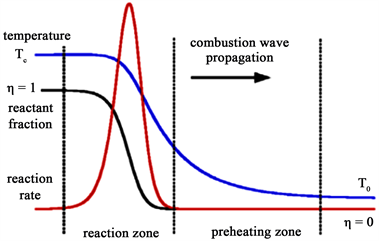Figure 1. Preheating and reaction zones in nanoscale thermite reaction

$c={c}_{1}\frac{y{\text{M}}_{1}}{y{\text{M}}_{1}+{\text{M}}_{2}}+{c}_{2}\frac{{\text{M}}_{2}}{y{\text{M}}_{1}+{\text{M}}_{2}}$ (5)

Al/Bi2O3反应单位质量放热焓由式(6)计算得到：

$H=\frac{\left({\text{2M}}_{1}+{\text{M}}_{2}\right){H}_{0}}{y{\text{M}}_{1}+{\text{M}}_{2}}×\frac{1}{2}\left(1+\frac{y}{2}-|\frac{y}{2}-1|\right)$ (6)

$\phi =-\frac{\partial \nu }{\partial t}=A\nu \mathrm{exp}\left(-\frac{{E}_{\text{a}}}{RT}\right)$ (7)

$V=\frac{\text{d}X}{\text{d}t}=\frac{\text{d}}{\text{d}t}{\int }_{0}^{x}\eta \text{d}x$ (8)

3. 结果与讨论

3.1. Al/Bi2O3燃烧特性数值计算结果与分析Table 1. Parameters in simulation of combustion characteristics of nanothermite Al/Bi2O3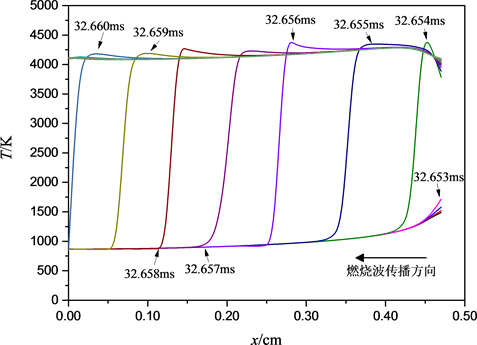Figure 2. Temperatures as a function of time and location in Al/Bi2O3 reaction

1) 燃烧前升温阶段(£32.653 m/s)：此阶段反应区温度较低，未达到点火温度，Al/Bi2O3化学反应速率较低，温度随着热源输入逐渐上升直至点火温度。

2) 燃烧反应阶段(32.654~32.660 m/s)：此阶段反应区温度达到了点火温度，Al/Bi2O3发生自持燃烧反应，形成燃烧波阵面，并放出大量热。反应放出的大量热量使反应区温度迅速上升，达到较高的温度输出。反应到达点火温度的时间点定义为点火(延迟)时间，用tini表示。

3) 燃烧后热力学局部热力学平衡阶段(>32.660 m/s)：此阶段随着反应进程接近于1，化学反应速率减小，放热减小，达到局部热力学平衡，温度基本不再发生变化。

Al/Bi2O3反应达到局部平衡时的计算温度平均值为4217 K，燃烧波传播计算平均速度为671.4 m/s，这两者的实际测量值分别为3592 K和617 m/s  。计算平衡温度高于实际测量温度值，这是因为实验和数值计算的点火热量输入值大小不同  。在实际反应过程中，当反应物升温至Al熔点(约933 K)时，Al熔化成液态，即与Bi2O3开始发生反应  。而在模拟计算过程中，由于通过式(4)化学反应速率的变化来控制燃烧过程的发生，温度高于1200 K时化学反应速率才发生突变跃迁，因此在计算中需要输入更多的热量，加热更长的时间，导致平衡温度高于实际测量值，且延迟时间也大于实际值。对于燃烧波速度，计算值与实验值基本吻合，同时也验证了计算模型的正确性。

Al/Bi2O3化学反应速率随时间、位置的变化关系如图3所示。图中每一条曲线表示该时刻下、不同位置处的化学反应速率。图3中曲线的最高值称为该时刻化学反应速率最大值，即最大反应速率，各曲线最大反应速率的平均值为平均最大反应速率，用φmax表示。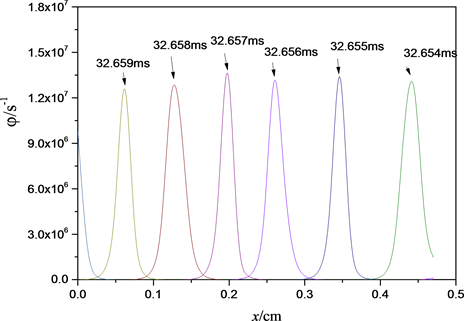Figure 3. Chemical reaction rates as a function of time and location in Al/Bi2O3 reaction

3.2. Al/Bi2O3燃烧特性影响因素

3.2.1. 配比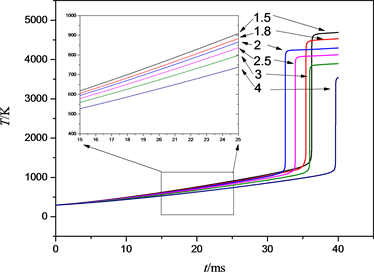Figure 4. Heating curves of the ignition with different mole ratio of Al/Bi2O3Figure 5. Ignition delay time as a function of mole ratio of Al/Bi2O3(a)(b)

Figure 6. Propagation velocities of combustion and maximum rates of reaction as functions of mole ratio of Al/Bi2O3. (a) Propagation velocities of combustion; (b) Maximum rates of reaction

3.2.2. 粒径

Al粉粒径分别为2 μm、100 nm、40 nm、24 nm的Al/Bi2O3复合物反应区温度、反应速率、燃烧波传播速度、点火时间随粒径的变化关系如表2所示，点火端的温升曲线如图7所示。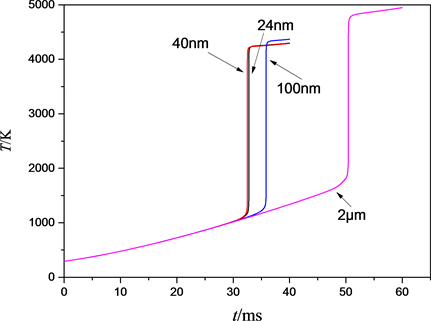Figure 7. Heating curves of the ignition with different particle sizeTable 2. Results in simulation of combustion characteristics of Al/Bi2O3 with different particle size

4. 结论

1) Al/Bi2O3温度计算结果表明，MIC材料在反应过程中经历了三个阶段，即点火前升温阶段、燃烧波传播阶段和局部热力学平衡阶段。

2) 由MIC材料反应影响因素计算结果可知，反应物配比为2的Al/Bi2O3复合材料反应性能最好，平衡温度可达4217 K，平均最大反应速率和燃烧波传播速度分别为1.36 × 107 s1和671.6 m/s。

3) 随着反应物粒径的减小，Al/Bi2O3的平衡温度逐渐减小，最大反应速率和燃烧波传播速度逐渐增大，反应物的活化能是影响反应点火时间的重要因素，且影响作用较化学反应的能量释放更明显。

Numerical Simulation of Combustion Char-acteristics of Nanothermite Al/Bi2O3[J]. 材料科学, 2017, 07(08): 706-715. http://dx.doi.org/10.12677/MS.2017.78093

1. 1. Zhou, X., Shen, R., Ye, Y., et al. (2011) Influence of Al/CuO Reactive Multilayer Films Additives on Exploding Foil Initiator. Journal of Applied Physics, 110, 094505.
https://doi.org/10.1063/1.3658617

2. 2. Sanders, V.E., Asay, B.W., Foley, T.J., et al. (2007) Reaction Propagation of Four Nanoscale Energetic Composites (Al/MoO3, Al/WO3, Al/CuO, and Al/Bi2O3). Journal of Propulsion and Power, 23, 707-714.
https://doi.org/10.2514/1.26089

3. 3. Piekiel, N.W., Zhou, L., Sullivan, K.T., et al. (2014) Initiation and Reaction in Al/Bi2O3 Nanothermites: Evidence for the Predominance of Condensed Phase Chemistry. Combustion Science & Technology, 186, 1209-1224.
https://doi.org/10.1080/00102202.2014.908858

4. 4. Stamatis, D., Ermolinem, A. and Dreizin, E.L. (2012) A Multi-Step Reaction Model for Ignition of Fully-Dense Al-CuO Nanocomposite Powders. Combustion Theory & Mod-elling, 16, 1-18.
https://doi.org/10.1080/13647830.2012.694480

5. 5. Baijot, V., Glavier, L., Ducere, J., et al. (2015) Modeling the Pressure Generation in Aluminum Based Thermites. Propellants, Explosives, Pyrotechnics, 40, 402-412.
https://doi.org/10.1002/prep.201400297

6. 6. Shaw, B.D., Pantoya, M.L. and Dikici, B. (2013) Detonation Models of Fast Combustion Waves in Nanoscale Al-MoO3 Bulk Powder Media. Combustion Theory & Modelling, 17, 25-39.
https://doi.org/10.1080/13647830.2012.721898

7. 7. Martirosyan, K.S., Zyskin, M., Jenkins, C.M., et al. (2014) Fluid Dynamic Modeling of Nano-Thermite Reactions. Journal of Applied Physics, 115, 104903.
https://doi.org/10.1063/1.4867936

8. 8. 王亚军, 李泽雪, 于海洋, 等. 亚稳态分子间复合物反应机理研究[J]. 化学进展, 2016, 28(11): 1689-1704.

9. 9. 杜志明, 冯长根, 杜霞, 等. 电热丝点火模型及其临界性[J]. 北京理工大学学报: 自然科学版, 1994(3): 264-268.

10. 10. 岑可法. 高等燃烧学[M]. 杭州: 浙江大学出版社, 2002.

11. 11. Kim, K. (2014) Computational Modeling of Combustion Wave in Nanoscale Thermite Reaction. International Journal of Chemical, Molecular, Nuclear, Materials and Metallurgical Engineering, 8, 685-688.

12. 12. Puszynski, J.A., Bulian, C.J. and Swiatkiewicz J.J. (2007) Processing and Ignition Characteristics of Aluminum-Bismuth Trioxide Nanothermite System. Journal of Propulsion & Power, 23, 698-706.
https://doi.org/10.2514/1.24915

13. 13. 张向宇. 实用化学手册[M]. 北京: 国防工业出版社, 2011.

14. 14. Aumann, C.E., Skofronick, G.L. and Martin, J.A. (1995) Oxidation Behavior of Aluminum Nanopowders. Journal of Vacuum Science & Technology B Microelectronics & Nanometer Structures, 13, 1178-1183.
https://doi.org/10.1116/1.588232

15. 15. Puszynski, J.A. (2009) Processing and Characterization of Alumi-num-Based Nanothermites. Journal of Thermal Analysis and Calorimetry, 96, 677-685.
https://doi.org/10.1007/s10973-009-0037-0

16. 16. Baijot, V., Mehdi, D.R., Rossi, C., et al. (2017) A Multi-Phase Micro-Kinetic Model for Simulating Aluminum Based Thermite Reactions. Combustion & Flame, 180, 10-19.
https://doi.org/10.1016/j.combustflame.2017.02.031

17. 17. 江自生. 亚稳态分子间复合物Al/Bi2O3的制备及性能研究[D]: [硕士学位论文]. 北京: 北京理工大学, 2016.

18. NOTES



*通讯作者。